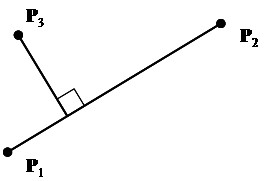ECE 564 Computer Vision Assignment 2

Submit HTML documentation on Isidore.

1. Using images from Isidore resources (CV2019-01-28.zip), find the effective focal length of the camera and the horizontal field of view. See calculating focal length.

The squares of the target board are 3 inches in size. Distances recorded should be feet and tenths of feet, not feet and inches. One of the images is a duplicate. The last image has a different efl (zoom must have shifted) and should be excluded. (See infotable

2. Construct a cube model, preferably including your picture on one of the faces. See example surface demo 2

3. Write a MATLAB script that uses ginput to get three arbitrary points and then produces the diagram shown below. As an extra challenge, superimpose this figure over an underlying image, using color to make the annotation stand out. Use axis equal to insure the same scale in both x and y directions.4. Given three arbitrary 3D points, write a MATLAB script that calculates the unit normal vector to the plane determined by those points. The vector should be oriented by the right-hand rule. If your fingers curl from point 1 to point 2 to point 3, then your thumb should point in the direction of the normal vector.

5. Write a MATLAB script that uses plot3 to draw a sprial similar to that shown below. Then show an orthographic projection. (see vis1.m)6. Assume a target point of [2, 4, 8], a camera location of [1, 0, 20], and an up vector of [0 0 1]. Find the matrix that converts world coordinates to camera coordinates. You should do this by hand (using MATLAB to do the work) and then by using the MATLAB camera specifications.

Maintained by John Loomis, last updated 4 Feb 2019